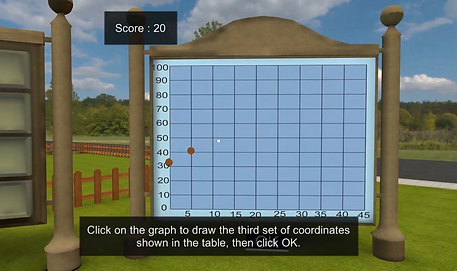### Linear Equations in Two Variables

###### Math

Solve the system of equations of two variables. A linear system of equations consists of two or more equations for calculating the common solution of the equation.